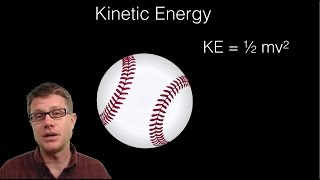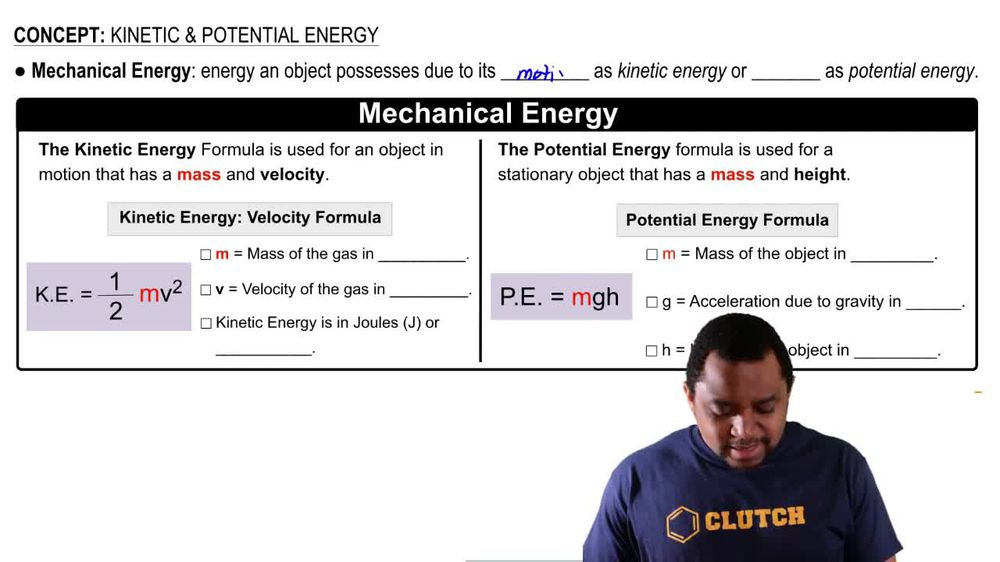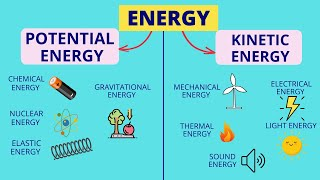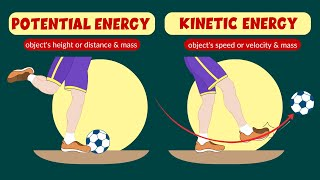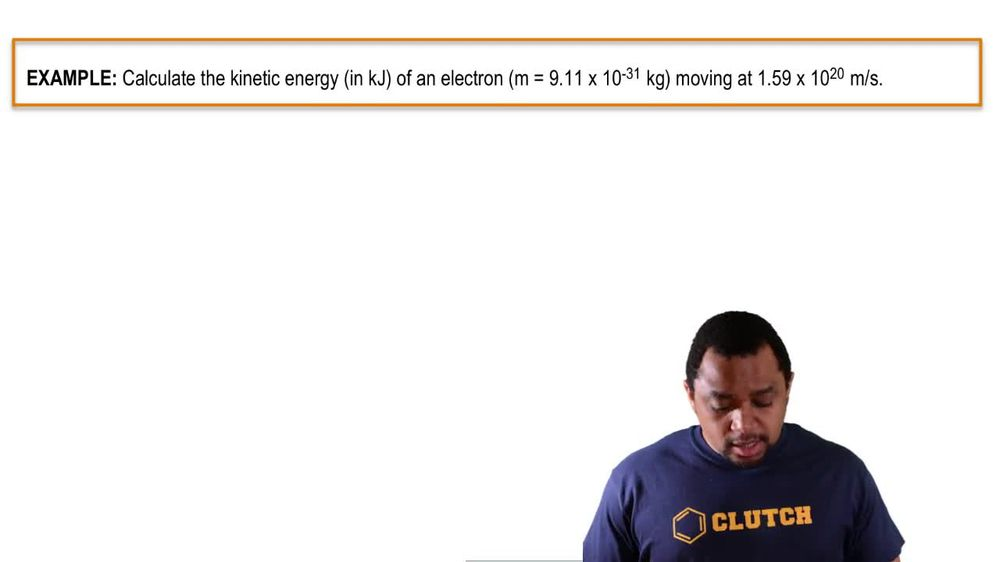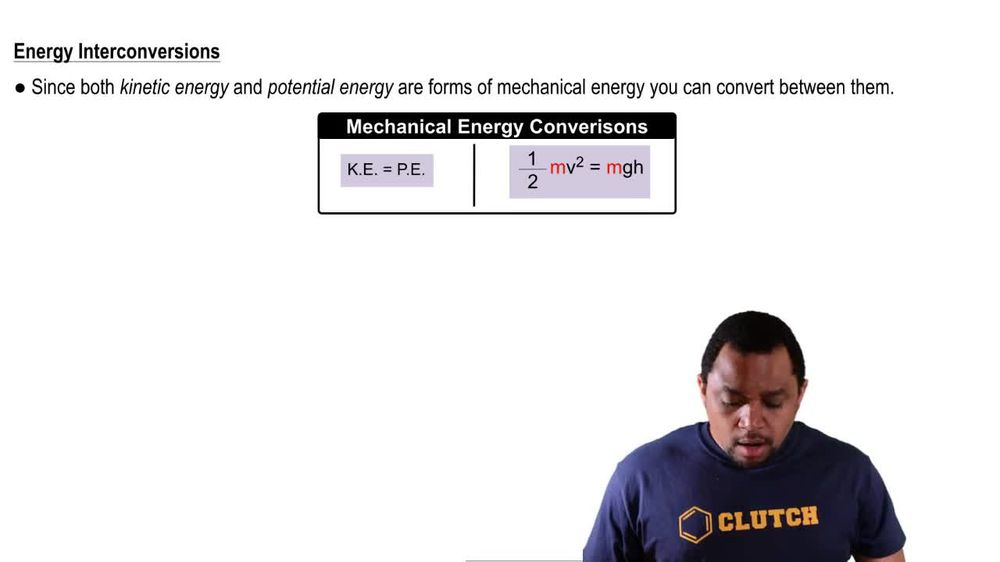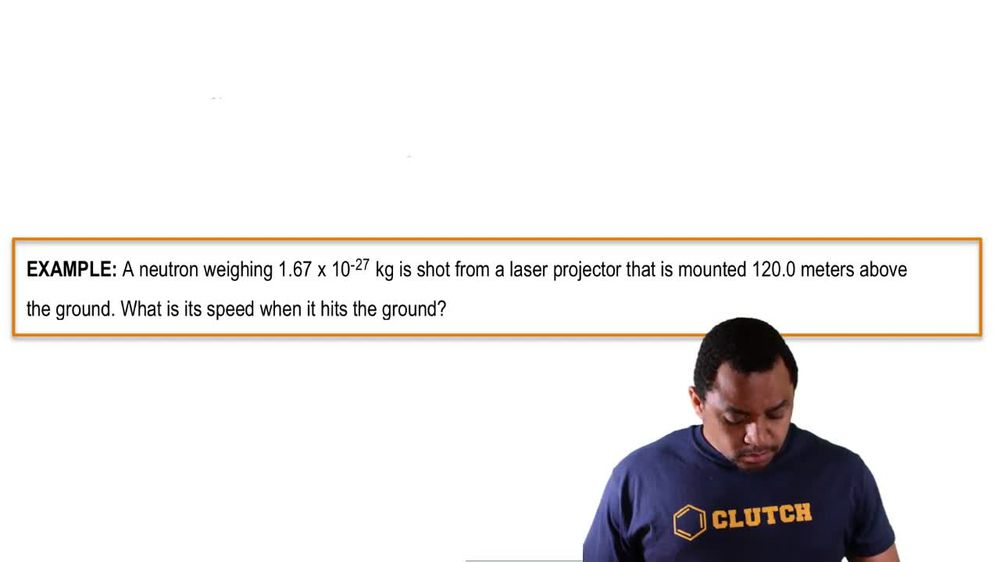Start typing, then use the up and down arrows to select an option from the list.
1. 8. Thermochemistry2. Kinetic & Potential Energy# Kinetic & Potential Energy

by Jules Bruno
173 views
1
1
1
now remember, Since kinetic energy and potential energy are forms of mechanical energy, you can convert between them. So that would mean that kinetic energy equals potential energy, which would mean that half times the mass of an object in kilograms times velocity squared equals the same mass of the object times gravity due to acceleration times, the height of the object and meters. So just remember, if you're given kinetic energy, there are ways to convert it into potential energy, and if you're given potential energy, you can convert it to kinetic energy.